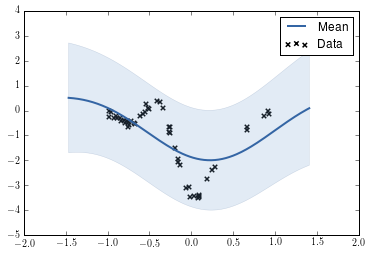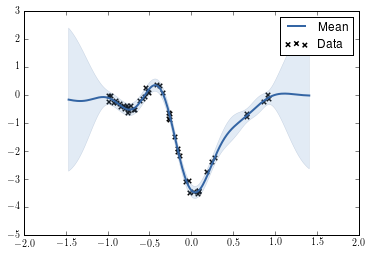In :
import GPy
from GPy.inference.optimization import Optimizer
%matplotlib inline

In :
import climin


In Paramz/GPy we can implement an own optimizer in a really simple way. We need to supply GPy with an implementation of the Optimizer class. The Optimizer has a name, which is the most important.

It also provides an opt() method, which opitmizes the result given an optimization function and a starting point.

In :
# Get the parameters for Rprop of climin:
climin.Rprop?

In :
class RProp(Optimizer):
# We want the optimizer to know some things in the Optimizer implementation:
def __init__(self, step_shrink=0.5, step_grow=1.2, min_step=1e-06, max_step=1, changes_max=0.1, *args, **kwargs):
super(RProp, self).__init__(*args, **kwargs)
self.opt_name = 'RProp (climin)'
self.step_shrink = step_shrink
self.step_grow = step_grow
self.min_step = min_step
self.max_step = max_step
self.changes_max = changes_max

def opt(self, x_init, f_fp=None, f=None, fp=None):
# We only need the gradient of the
assert not fp is None

# Do the optimization, giving previously stored parameters
opt = climin.rprop.Rprop(x_init, fp,
step_shrink=self.step_shrink, step_grow=self.step_grow,
min_step=self.min_step, max_step=self.max_step,
changes_max=self.changes_max)

# Get the optimized state and transform it into Paramz readable format by setting
# values on this object:
# Important ones are x_opt and status:
for info in opt:
if info['n_iter']>=self.max_iters:
self.x_opt =  opt.wrt
self.status = 'maximum number of function evaluations exceeded'
break


This is all we need, GPy/Paramz will handle the rest for you : )

In :
m = GPy.examples.regression.toy_rbf_1d_50(optimize=False, plot=False)


This is the model plot before optimization:

In :
m

Out:

Model: GP regression
Objective: 71.7157240818
Number of Parameters: 3
Number of Optimization Parameters: 3

GP_regression. valueconstraintspriors
rbf.variance 1.0 +ve
rbf.lengthscale 1.0 +ve
Gaussian_noise.variance 1.0 +ve
In :
m.plot()

Out:
<matplotlib.axes._subplots.AxesSubplot at 0x7f2be4307d90>In :
m.optimize(RProp(), messages=1)


And then the optimized state after running RProp:

In :
m

Out:

Model: GP regression
Objective: -15.5366629083
Number of Parameters: 3
Number of Optimization Parameters: 3
m.plot()

<matplotlib.axes._subplots.AxesSubplot at 0x7f2be42fd050>